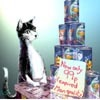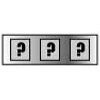# Search by Topic

#### Resources tagged with Triangle numbers similar to Triangular Triples:

Filter by: Content type:
Age range:
Challenge level:

### There are 20 results

Broad Topics > Numbers and the Number System > Triangle numbers### Triangular Triples

##### Age 14 to 16 Challenge Level:

Show that 8778, 10296 and 13530 are three triangular numbers and that they form a Pythagorean triple.### Take Ten Sticks

##### Age 11 to 16 Challenge Level:

Take ten sticks in heaps any way you like. Make a new heap using one from each of the heaps. By repeating that process could the arrangement 7 - 1 - 1 - 1 ever turn up, except by starting with it?### Satisfying Statements

##### Age 11 to 14 Challenge Level:

Can you find any two-digit numbers that satisfy all of these statements?### Iff

##### Age 14 to 18 Challenge Level:### Slick Summing

##### Age 14 to 16 Challenge Level:

Watch the video to see how Charlie works out the sum. Can you adapt his method?### Factors and Multiples Puzzle

##### Age 11 to 14 Challenge Level:

Using your knowledge of the properties of numbers, can you fill all the squares on the board?### Series Sums

##### Age 14 to 16 Challenge Level:

Let S1 = 1 , S2 = 2 + 3, S3 = 4 + 5 + 6 ,........ Calculate S17.### Mystic Rose

##### Age 14 to 16 Challenge Level:

Use the animation to help you work out how many lines are needed to draw mystic roses of different sizes.### Triangles Within Squares

##### Age 14 to 16 Challenge Level:

Can you find a rule which relates triangular numbers to square numbers?### Route to Infinity

##### Age 11 to 14 Challenge Level:

Can you describe this route to infinity? Where will the arrows take you next?### Human Food

##### Age 7 to 14 Challenge Level:

Sam displays cans in 3 triangular stacks. With the same number he could make one large triangular stack or stack them all in a square based pyramid. How many cans are there how were they arranged?### Picturing Triangular Numbers

##### Age 11 to 14 Challenge Level:

Triangular numbers can be represented by a triangular array of squares. What do you notice about the sum of identical triangle numbers?### Hot Pursuit

##### Age 11 to 14 Challenge Level:

I added together the first 'n' positive integers and found that my answer was a 3 digit number in which all the digits were the same...### Sam Again

##### Age 11 to 14 Challenge Level:

Here is a collection of puzzles about Sam's shop sent in by club members. Perhaps you can make up more puzzles, find formulas or find general methods.### Triangles Within Pentagons

##### Age 14 to 16 Challenge Level:

Show that all pentagonal numbers are one third of a triangular number.### Reasonable Algebra

##### Age 14 to 16 Challenge Level:

Use algebra to reason why 16 and 32 are impossible to create as the sum of consecutive numbers.### Forgotten Number

##### Age 11 to 14 Challenge Level:

I have forgotten the number of the combination of the lock on my briefcase. I did have a method for remembering it...### Handshakes

##### Age 11 to 14 Challenge Level:

Can you find an efficient method to work out how many handshakes there would be if hundreds of people met?### Triangle Numbers

##### Age 11 to 14 Challenge Level:

Take a look at the multiplication square. The first eleven triangle numbers have been identified. Can you see a pattern? Does the pattern continue?### Triangles Within Triangles

##### Age 14 to 16 Challenge Level:

Can you find a rule which connects consecutive triangular numbers?